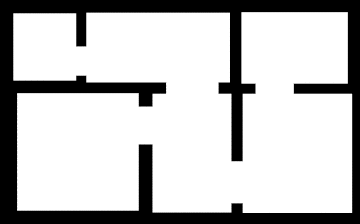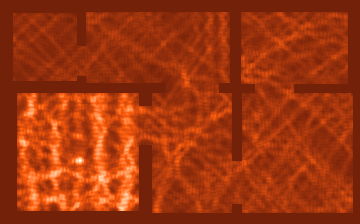# Where's my WIFI signal ?¶

## Solving the Helmhotz equation to find the good spots in your home¶

The great blog article http://jasmcole.com/2014/08/25/helmhurts/ explains how to apply the Maxwell equations, and in particular their smaller sibling the Helmholtz equations, to calculate the propagation of WIFI signals.

What follows is an attempt to use Julia to go through the whole process described in the blog entry.

The only packages necessary are Images, ImageView and Color that will allow us to import the floor plan at the start and produce the amplitude of the signal field at the end. All the other functions used (including the sparse matrix operations) are already provided by the base library of Julia

### Setting the up the data¶

We'll start first by importing a floor plan, in that case 320x224 bitmap image that I produced with an image editor, but you can of course create your own image of your own place (or an imaginary one).

In :
using Color
using Images
using ImageView

In :
img = imread("/home/fred/Images/plan.png")

Out:In :
size(img)

Out:
(4,360,224)

Here we have an image structure containing an array of three dimensions, 2 spatial dimension and 1 color dimension of size 4 for each of the 3 colors (Red, Green and Blue) plus an alpha channel. This is a color model akso known as RGBA. For the purpose of the simulation we only need to know if a given point is air or concrete.

Let's extract that material information from one of the color planes :

In :
plan = squeeze(img.data[2,:,:],1);


And set the necessary variables :

In :
dimx, dimy = size(plan)  # spatial dimensions
fsize = length(plan)     # full size of the problem
δ = 0.03                 # spatial resolution of each pixel

Out:
0.03

Note that the spatial resolution is given by the actual size of the appartment divided by the number of pixel making the plan picture. Two important considerations come into play here :

1. Beware of not making the problem size (the number of pixels) too important, I found on my setup that you can go quickly from a problem that can be solved in seconds (100 x 100) to a never ending calculation or one that throws out of memory exceptions (1000 x 1000 in my case).
2. You can't on the other hand keep the pixel count low by increasing the spatial step δ (i.e. decreasing the resolution) : when it gets too close to the wavelength (about 10 cm for WIFI signals) the mesh becomes too coarse to give meaningful results.

Here, with a spatial resolution of 3 cm we should be safe for the resolution while still simulating a normally sized appartment of about 10m x 6m

In :
η_air = 1.                 # refraction index for air
η_concrete = 2.55 - 0.01im  # refraction index for concrete
# the imaginary part conveys the absorption

λ = 0.12                  # for a 2.5 GHz signal, wavelength is ~ 12cm
k = 2π / λ                # k is the wavenumber

Out:
52.35987755982989

We'll now create the Complex Array containing the material information :

In :
μ = similar(plan, Complex)
μ[plan .!= 0] = (k / η_air)^2
μ[plan .== 0] = (k / η_concrete)^2;


Note that we directly calculate the $\left( \frac{k}{n} \right) ^2$ value appearing in the Helmholtz equation below.

### Solving the equation¶

The Helmholtz equation for the amplitude of the electric field is : $$\Delta A + \left( \frac{k}{n} \right) ^2 A= f$$ $\Delta$ being the Laplacian operator, $A$ the amplitude field and $f$ the wave source that will be equal to zero everywhere except where the antenna is.

The first step is to rephrase that problem in one of the ways (there are several) a computer can tackle it : by discretizing the space and using finite differences.

In that framework the Laplacian can be approximated by the neighboring values, giving us : $$\frac{A(x+δ,y) - 2 A(x,y) + A(x-δ,y)}{δ^2}+\frac{A(x,y+δ) - 2 A(x,y) + A(x,y-δ)}{δ^2}+ \left( \frac{k}{n} \right) ^2 A(x,y) = f(x,y)$$

We have here a linear combination of elements of A equal to elements of f. In others words an ordinary system of linear equations that computers are good at solving.

There is however a roadblock : that system has as many equations as there are values in A : 360 x 224 = 80.640. The matrix describing the problem would occupy an huge amount of memory if stored as a dense array : 80.640 x 80.640 x 8 x 2 (don't forget these are complex numbers) = 96 Terabytes !

This memory would be mostly wasted though as the matrix is almost empty (only 5 elements appear in each equation not the whole 80.640). Sparses matrices are the adapted structure in these situations as they only store the non zero elements in memory. Julia provides a sparse matrix type on which most dense matrix methods can apply.

Let's now build the three vectors describing the non zero elements of the sparse matrix S (the sub2ind() function relates the (x,y) positions in the real space to an index in the matrix):

In :
xs = Array(Int, 5*dimx*dimy)
ys = Array(Int, 5*dimx*dimy)
vs = Array(Complex, 5*dimx*dimy)
i = 1
for x in 1:dimx, y in 1:dimy  #  x=1; y=1
xm = (x+dimx-2) % dimx + 1
xp =          x % dimx + 1
ym = (y+dimy-2) % dimy + 1
yp =          y % dimy + 1

xs[i] = sub2ind((dimx,dimy), x, y); ys[i] = sub2ind((dimx,dimy),  x,  y); vs[i] = μ[x,y] - 2*δ^-2
i += 1
xs[i] = sub2ind((dimx,dimy), x, y); ys[i] = sub2ind((dimx,dimy), xp,  y); vs[i] = δ^-2
i += 1
xs[i] = sub2ind((dimx,dimy), x, y); ys[i] = sub2ind((dimx,dimy), xm,  y); vs[i] = δ^-2
i += 1
xs[i] = sub2ind((dimx,dimy), x, y); ys[i] = sub2ind((dimx,dimy),  x, yp); vs[i] = δ^-2
i += 1
xs[i] = sub2ind((dimx,dimy), x, y); ys[i] = sub2ind((dimx,dimy),  x, ym); vs[i] = δ^-2
i += 1
end


We can now declare S by calling the sparse() function :

In :
S = sparse(xs, ys, vs, fsize, fsize);


There is only the emiter field $f$ left to define :

In :
f = fill(0. + 0.im, (dimx, dimy))
f[80:82, 160:162] = 1.0;                  # our Wifi emitter antenna will be there;


Now, with the above notations, we have the linear system $S.A=f$ to solve for A :

In :
A = reshape(S \ vec(f), dimx, dimy);


A contains the amplitude field we are looking for in the real part of the complex number. Since the relevant physical value is rather the Energy received not the amplitude, we'll calculate the square of the amplitude.

In :
E = real(A) .* real(A);


And since the receiving antennas of laptops and smartphones have a size of at least 10cm, we'll convolve the energy field to have a more accurate representation of the received signal strength

In :
E = conv2(E, ones(5,5)/25 )[3:end-2, 3:end-2];


### Plotting the result¶

We can now convert the E field back to an image to visualize where the good spots are for WIFI reception in our appartment.

First we'll translate the energy field to 1-100 interger scale :

In :
Ei = iround(min(100, max(1, (int( 1 .+ 100 .* (E .- minimum(E)) / (maximum(E) - minimum(E)) ) ))));


Then we'll create a color scale using the colormap() function of the Color package :

In :
cm = reverse(colormap("oranges"));


And set the walls to the darkest color :

In :
Ei[plan .== 0] = 1;


The last step is to create a color image by picking the RGB components of the color scale :

In :
field = Array(Float64, (dimx, dimy, 3))
field[:,:,1] = [ cm[Ei[i]].r for i in 1:fsize ]
field[:,:,2] = [ cm[Ei[i]].g for i in 1:fsize ]
field[:,:,3] = [ cm[Ei[i]].b for i in 1:fsize ]
fim = colorim(permutedims(field, [2, 1, 3]))

Out: